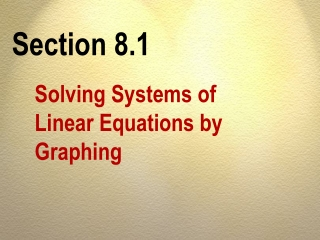Download PresentationSection 8.1

# Section 8.1

Download Presentation## Section 8.1

- - - - - - - - - - - - - - - - - - - - - - - - - - - E N D - - - - - - - - - - - - - - - - - - - - - - - - - - -
##### Presentation Transcript

1. Section 8.1 Solving Systems of Linear Equations by Graphing

2. Solve a system of two equations in two variables by graphing. A OBJECTIVES

3. B OBJECTIVES Determine whether a system of equations is consistent, inconsistent, or dependent.

4. C OBJECTIVES Solve an application.

5. SOLVING SYSTEMS OF SIMULTANEOUS EQUATIONS There are three possible solutions.

6. POSSIBLE SOLUTION 1 Consistent and independent equations: The graphs of the equations intersect at one point, whose coordinates give the solution of the system.

7. POSSIBLE SOLUTION 2 Inconsistent equations: The graphs of the equations are parallel lines; there is no solution for the system

8. POSSIBLE SOLUTION 3 Dependent equations: The graphs of the equations coincide (are the same). There are infinitely many solutions for the system.

9. COMPARISONS Intersecting Lines Have different slopes

10. COMPARISONS Intersecting Lines Have one solution

11. COMPARISONS Intersecting Lines Form a consistent system

12. COMPARISONS Parallel Lines Have the same slopes

13. COMPARISONS Parallel Lines Have different y-intercepts

14. COMPARISONS Parallel Lines Have no solution

15. COMPARISONS Parallel Lines Form inconsistent systems

16. COMPARISONS Coinciding Lines Have the same slope

17. COMPARISONS Coinciding Lines Have the samey-intercept

18. COMPARISONS Coinciding Lines Have infinite solutions

19. COMPARISONS Coinciding Lines Form a dependent system

20. Chapter 8 Solving Systems of Linear Equations and Inequalities Section 8.1

21. Chapter 8 Solving Systems of Linear Equations and Inequalities Section 8.1Exercise #1

22. Use the graphical method to solve the system.

23. x y0 24 0 Use the graphical method to solve the system.

24. x y0 04 2 Use the graphical method to solve the system.

25. Chapter 8 Solving Systems of Linear Equations and Inequalities Section 8.1Exercise #2

26. Use the graphical method to solve the system.

27. x y0 2–1 0 Use the graphical method to solve the system.

28. x y0 4–2 0 Use the graphical method to solve the system. Inconsistent:No Solution.

29. Section 8.2 Solving Systems of Equations by Substitution

30. Solve a system of equations in two variables. A OBJECTIVES

31. B OBJECTIVES Determine whether a system of equations is consistent, inconsistent, or dependent.

32. C OBJECTIVES Solve an application.

33. PROCEDURE: Solving a system of equations by the Substitution Method. 1. Solve one of the equations for x or y.

34. PROCEDURE: Solving a system of equations by the Substitution Method. Substitute the resulting expression into the other equation.

35. PROCEDURE: Solving a system of equations by the Substitution Method. 3. Solve the new equation for the variable.

36. PROCEDURE: Solving a system of equations by the Substitution Method. 4. Substitute the value of the variable and solve to get the value for the second variable.

37. PROCEDURE: Solving a system of equations by the Substitution Method. 5. Check the solution by substituting the numerical values of the variables in both equations.

38. Chapter 8 Solving Systems of Linear Equations and Inequalities Section 8.2Exercise #3

39. Use the method of substitution to solve the system (if possible). No Solution (inconsistent)

40. Chapter 8 Solving Systems of Linear Equations and Inequalities Section 8.2Exercise #4

41. Use the method of substitution to solve the system (if possible). Dependent (infinitely many solutions).

42. Section 8.3 Solving Systems of Equations by Elimination

43. Solve a system of equations in two variables. A OBJECTIVES

44. B OBJECTIVES Determine whether a system of equations is consistent, inconsistent, or dependent.

45. C OBJECTIVES Solve an application.

46. ELIMINATION METHOD One or both equations in a system of simultaneous equations can be multiplied (or divided) by any nonzero number to obtain an equivalent system.

47. ELIMINATION METHOD In the equivalent system, the coefficients of x (or y) are opposites, thus eliminatingx or y when the equations are added.

48. Chapter 8 Solving Systems of Linear Equations and Inequalities Section 8.3Exercise #5